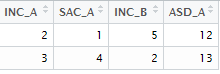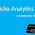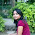# R : Keep / Drop Columns from Data Frame

The article below explains how to keep or drop variables (columns) from data frame. In R, there are multiple ways to select or drop column.
Create a sample data frame
The following code creates a sample data frame that is used for demonstration.
set.seed(456)
mydata <- data.frame(a=letters[1:5], x=runif(5,10,50), y=sample(5), z=rnorm(5))Sample Data
R : Delete column by name
Method I :
The most easiest way to drop columns is by using subset() function. In the code below, we are telling R to drop variables x and z. The '-' sign indicates dropping variables. Make sure the variable names would NOT be specified in quotes when using subset() function.
df = subset(mydata, select = -c(x,z) )
```  a y
1 a 2
2 b 1
3 c 4
4 d 3
5 e 5```
Method II :
In this method, we are creating a character vector named drop in which we are storing column names x and z. Later we are telling R to select all the variables except the column names specified in the vector drop. The function names() returns all the column names and the '!' sign indicates negation.
drop <- c("x","z")
df = mydata[,!(names(mydata) %in% drop)]
It can also be written like :  df = mydata[,!(names(mydata) %in% c("x","z"))]
R : Drop columns by column index numbers
It's easier to remove variables by their position number. All you just need to do is to mention the column index number. In the following code, we are telling R to drop variables that are positioned at first column, third and fourth columns. The minus sign is to drop variables.
df <- mydata[ -c(1,3:4) ]
```         x
1 13.58206
2 18.42049
3 39.31821
4 44.08534
5 41.53592
```
R : Keep column by name
Method I :
In this section, we are retaining variables x and z.
keeps <- c("x","z")
df = mydata[keeps]
The above code is equivalent to df = mydata[c("x","z")]
Method II :
We can keep variables with subset() function.
df = subset(mydata, select = c(x,z))
Keep columns by column index number
In this case, we are telling R to keep only variables that are placed at second and fourth position.
df <- mydata[c(2,4)]
Keep or Delete columns with dplyr package
In R, the dplyr package is one of the most popular package for data manipulation. It makes data wrangling easy. You can install package by using the command below -
install.packages("dplyr")
1. How to delete first, third and fourth column
library(dplyr)
mydata2 = select(mydata, -1, -3:-4)
2. How to delete columns a, x and y
This can be written in three ways -
mydata2 = select(mydata, -a, -x, -y)
mydata2 = select(mydata, -c(a, x, y))
mydata2 = select(mydata, -a:-y)
3. How to keep columns a, y and z
mydata2 = select(mydata, a, y:z)
Keep / Drop Columns by Name Pattern
The code below creates data for 4 variables named as follows :
INC_A   SAC_A   INC_B   ASD_A
INC_A SAC_A INC_B ASD_A
2 1 5 12
3 4 2 13Keep / Drop Columns by pattern
Keeping columns whose name starts with "INC"
mydata1 = mydata[,grepl("^INC",names(mydata))]
The grepl() function is used to search for matches to a pattern. In this case, it is searching "INC" at starting in the column names of data frame mydata. It returns INC_A and INC_B.
Dropping columns whose name starts with "INC"

mydata2 = mydata[,!grepl("^INC",names(mydata))]
Keeping columns whose name contain "_A" at the end
The "\$" is used to search for the sub-strings at the end of string. It returns INC_A, SAC_A and ASD_A.
mydata12 = mydata[,grepl("_A\$",names(mydata))]
Dropping columns whose name contain "_A" at the end
mydata22 = mydata[,!grepl("_A\$",names(mydata))]
Keeping columns whose name contain the letter "S"
mydata32 = mydata[,grepl("*S",names(mydata))]
The same logic can be applied to a word as well if you wish to find out columns containing a particular word. In the example below, we are trying to keep columns where it contains `C_A` and creates a new dataframe for the retained columns.
```mydata320 = mydata[,grepl("*C_A",names(mydata))]
```
Dropping columns whose name contain the letter "S"
mydata33 = mydata[,!grepl("*S",names(mydata))]
Drop columns having more than 50% missing values
I have created a dummy data frame which includes several missing or blank values for illustration.
```df= data.frame(x=c(1,2,3,NA,NA), y=c(5,NA,3,NA,NA), Z=c(5,3,3,4,NA))
```
```  x  y  Z
1  1  5  5
2  2 NA  3
3  3  3  3
4 NA NA  4
5 NA NA NA
```
`sapply` function is an alternative of `for loop`. It runs a built-in or user-defined function on each column of data frame. `sapply(df, function(x) mean(is.na(x)))` returns percentage of missing values in each column in your dataframe.
```df = df[,!sapply(df, function(x) mean(is.na(x)))>0.5]
```
The above program removed column Y as it contains 60% missing values more than our threshold of 50%. Output is given below.
```  x  Z
1  1  5
2  2  3
3  3  3
4 NA  4
5 NA NA
```
R Function : Keep / Drop Column Function
The following program automates keeping or dropping columns from a data frame.
```KeepDrop = function(data=df,cols="var",newdata=df2,drop=1) {
# Double Quote Output Dataset Name
t = deparse(substitute(newdata))
# Drop Columns
if(drop == 1){
newdata = data [ , !(names(data) %in% scan(textConnection(cols), what="", sep=" "))]}
# Keep Columns
else {
newdata = data [ , names(data) %in% scan(textConnection(cols), what="", sep=" ")]}
assign(t, newdata, .GlobalEnv)
}
```
How to use the above function
To keep variables 'a' and 'x', use the code below. The drop = 0 implies keeping variables that are specified in the parameter "cols". The parameter "data" refers to input data frame. "cols" refer to the variables you want to keep / remove. "newdata" refers to the output data frame.
KeepDrop(data=mydata,cols="a x", newdata=dt, drop=0)

To drop variables, use the code below. The drop = 1 implies removing variables which are defined in the second parameter of the function.
KeepDrop(data=mydata,cols="a x", newdata=dt, drop=1)Share
Related PostsDeepanshu founded ListenData with a simple objective - Make analytics easy to understand and follow. He has over 10 years of experience in data science. During his tenure, he has worked with global clients in various domains like Banking, Insurance, Private Equity, Telecom and Human Resource.

22 Responses to "R : Keep / Drop Columns from Data Frame"
1.df =df[,-c(21,6,5,7,15,2,3)]

this can work too
these no. are the column number

2.Great summary, thank you!

1.Thank you for stopping by my blog!

3.Best computer science site ever, Thank you from Quito Ecuador.

1.4.Good examples !!!!

1.5.It was nice and easy to understand

6.How to remove a columns which have more than 75% missing values using which()

1.I have added desired details in article. Thanks!

7.If I want to remove all the cells containing "other" From a given data frame. How to create this dataset??

8.I am trying to remove columns where all variables are below a threshold of 5. Any advice?

1.What do you mean by "variables are below a threshold of 5"? Is it based on the number of rows in a variable?

9.Very well explained. Now I am able to do data preprocessing on my own :-). Thank You for sharing.

10.Hi I want to ask something, I want to delete all the values ​​in a column from all the data I do not want. More precisely, I will delete all values ​​of that column in the data, how can I do that?

1.Loans\$LoanID <- NA

11.That didn't work. When I did the keeps portion of the code, R is giving me an undefined columns selected error. Please help me on this. The assignment is due this sunday so would appreciate a response by tomorrow.

12.Thanks! This helped me out

13.I think that there is an error in your example titled "R : Delete column by name: Method II". Your code is: ```df = mydata[,!(names(mydata) %in% drop)]``` but I think it should be ```df = mydata[,!!(names(mydata) %in% drop)]``` I tried it the first way and couldn't get it to work but then after searching around on other sites found a suggestion that !! is correct. Maybe this is not always true but it helped me.

1.Actually, I was able to make both methods work!

14.Quick question... how to user subset(eastTable, select = -c(GB, PA/G, SRS)) and make it work for the column name "PA/G"?
15.